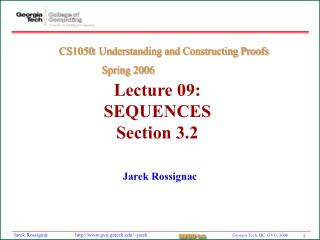# Lecture 09: SEQUENCES Section 3.2 - PowerPoint PPT PresentationDownload PresentationLecture 09: SEQUENCES Section 3.2

Lecture 09: SEQUENCES Section 3.2Download Presentation## Lecture 09: SEQUENCES Section 3.2

- - - - - - - - - - - - - - - - - - - - - - - - - - - E N D - - - - - - - - - - - - - - - - - - - - - - - - - - -
##### Presentation Transcript

1. Lecture 09: SEQUENCESSection 3.2 CS1050: Understanding and Constructing Proofs Spring 2006 Jarek Rossignac

2. Lecture Objectives • Analyze/evaluate sequences

3. What is a sequence? A function that maps an element n in the set {0,1,2…} or {1,2,3…} into the term an in an ordered set S. Notation {an} describes the sequence. For example {1/n} is {1, 1/2, 1/3,…}

4. What is an arithmetic progression? {a+nd} a = initial term d = common difference Examples: {1, 3, 5, 7…} {(–1)n} = ?

5. What is a geometric progression? {arn} a = initial term d = common ratio Examples: {1, 2, 4, 8…} {(–1)n} = ?

6. How to find the formula for a sequence • 1, –1/2, 1/4, –1/8… an=? • 1, 2, 2, 3, 3, 3, 4, 4, 4, 4, …? • 1, 8, 27… an=? • 3, 9, 27, 81… an=? • 1, 7, 25, 79, 241… an=?

7. Next term?

8. How to compute sums of sequences? ∑k=0n(k) = (n+1)n/2 ∑k=0n(rk) = (rn+1–1)/(r–1) for r≠1 ∑k=0(xk) = 1/(1–x) for |x|<1

9. What is a countable set? • A and B have the same cardinality if there is a bijection between them. • A set is countable is it has the same cardinality as the set of positive integers. • Positive rational numbers are countable • Real numbers are not

10. Assigned Homework • Page 236-237: 9g and 28

11. Assigned Project